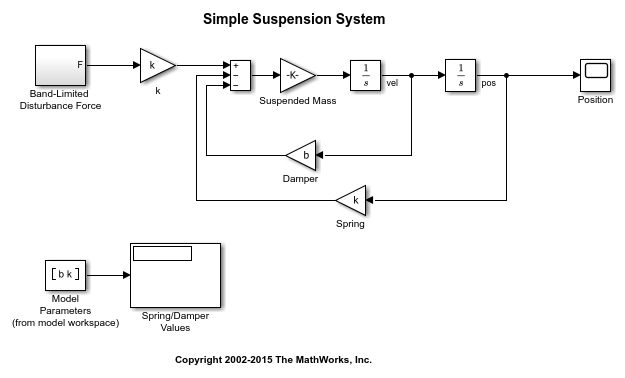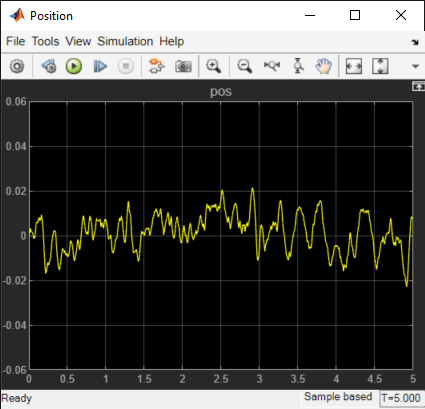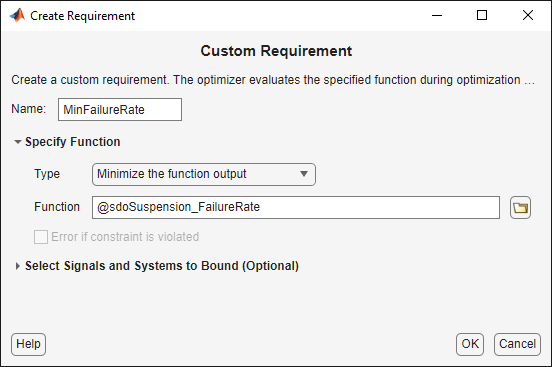# Design Optimization to Meet Frequency-Domain Requirements (GUI)

This example shows how to tune model parameters to meet frequency-domain requirements using the Response Optimizer app.

This example requires Simulink® Control Design™.

### Suspension Model

```open_system('sdoSimpleSuspension') ```Mass-spring-damper models represent simple suspension systems and for this example you tune the system to meet typical suspension requirements. The model implements the second order system representing a mass-spring-damper using Simulink blocks and includes:

• a `Mass` gain block parameterized by the total suspended mass, `m0+mLoad`. The total mass is the sum of a nominal mass,|m0|, and a variable load mass, `mLoad`.

• a `Damper` gain block parameterized by the damping coefficient, `b`.

• a `Spring` gain block parameterized by the spring constant, `k`.

• two integrator blocks to compute the mass velocity and position.

• a `Band-Limited Disturbance Force` block applying a disturbance force to the mass. The disturbance force is assumed to be band-limited white noise.

Simulate the model to view the system response to the applied disturbance force.### Design Problem

The initial system has a bandwidth that is too high. This can be seen from the spiky position signal. You tune the spring and damper values to meet the following requirements:

• The -3dB system bandwidth must not exceed 10 rad/s.

• The damping ratio of the system must be less than 1/sqrt(2). This ensures that no frequencies in pass band are amplified by the system.

• Minimize the expected failure rate of the system. The expected failure rate is described by a Weibull distribution dependent on the mass, spring, and damper values.

• These requirements must all be satisfied as the load mass ranges from 0 to 20.

### Open the Response Optimizer

In the Apps tab, click Response Optimizer under Control Systems.

### Specify Design Variables

In the Design Variables Set list, select New. Add the `b` and `k` model variables to the design variable set.

• Specify the `Minimum` and `Maximum` values for the `b` variable as 100 and 10000 respectively.

• Specify the `Minimum` and `Maximum` values for the `k` variable as 10000 and 100000 respectively.Click OK. A new variable, `DesignVars`, appears in the Response Optimizer browser.

In the Uncertain Variables Set list, select New. Add the `mLoad` variable to the uncertain variables set.

• Specify the `Uncertain Values` value for the `mLoad` variable as [10 15 20]Click OK. A new variable, `UncVars`, appears in the Response Optimizer browser.

### Specify Linear Analysis Input/Output Points

Specify the input/output points defining the linear system used to compute the bandwidth and damping ratio.

To specify the input/output points:

• In the New list, select Linearization I/Os.

• In the Simulink model, click the signal at the output of the `Band-Limited Disturbance Force` block. The Create linearization I/O set dialog box is updated and the chosen signal appears in it.

• In the Create linearization I/O dialog box, select the signal and click Add.

• In the Configuration list for the selected signal, choose Input Perturbation to specify it as an input signal.• Similarly, add the pos signal from the Simulink model. Specify this signal as an output. In the Configuration list, select Output Measurement.• Click OK. A new variable, `IOs`, appears in the Response Optimizer browser.

### Add Bandwidth and Damping-Ratio Requirements

Tune the spring and damper values to satisfy bandwidth and damping ratio requirements.

To specify the bandwidth requirement:

• Open a dialog to specify bounds on the Bode magnitude. In the New list, select Bode Magnitude.

• Specify the requirement name as `Bandwidth`.

• Specify the edge start frequency and magnitude as 10 rad/s and -3db, respectively.

• Specify the edge end frequency and magnitude as 100 rad/s and -3db, respectively.

• Specify the input/output set to which the requirement applies by clicking Select Systems to Bound. Select the `IOs` check box.• Click OK. A new requirement, `Bandwidth`, appears in the Response Optimizer browser and a graphical view of the bandwidth requirement is automatically created.

To specify the damping ratio requirement:

• Open a dialog to specify bounds on the damping ratio. In the New list, select Damping Ratio.

• Specify the damping ratio bound value as 0.7071.

• Specify the input/output set to which the requirement applies by clicking Select Systems to Bound. Select the `IOs` check box.• Click OK. A new requirement, `DampingRatio`, appears in the Response Optimizer browser and a graphical view of the damping ratio requirement is automatically created.

Tune the spring and damper values to minimize the expected failure rate over a lifetime of 100e3 miles. The failure rate is computed using a Weibull distribution on the damping ratio of the system. As the damping ratio increases the failure rate is expected to increase.

Specify the reliability requirement as a custom requirement:

• Open a dialog box to specify the custom requirements. In the New list, select Custom Requirement.

• Specify the custom requirement name as `MinFailureRate`.

• In the Specify Function area, select Minimize the function output from the Type list.

• Specify the function as @sdoSuspension_FailureRate.• Click OK. A new requirement, `MinFailureRate`, appears in the Response Optimizer browser and a graphical view of the custom requirement is automatically created.

The @sdoSuspension_FailureRate function returns expected failure rate for a lifetime of 100e3 miles.

```type sdoSuspension_FailureRate ```
```function pFailure = sdoSuspension_FailureRate(data) %SDOSUSPENDION_FAILURERATE % % The sdoSuspension_FailureRate function is used to define a custom % requirement that can be used in the graphical SDTOOL environment. % % The |data| input argument is a structure with fields containing the % design variable values chosen by the optimizer. % % The |pFailure| return argument is the failure rate to be minimized by the % SDOTOOL optimization solver. The failure rate is given by a Weibull % distribution that is a function of the mass, spring and damper values. % The design minimizes the failure rate for a 100e3 mile lifetime. % % Copyright 2012 The MathWorks, Inc. %Get the spring and damper design values allVarNames = {data.DesignVars.Name}; idx = strcmp(allVarNames,'k'); k = data.DesignVars(idx).Value; idx = strcmp(allVarNames,'b'); b = data.DesignVars(idx).Value; %Get the nominal mass from the model workspace wksp = get_param('sdoSimpleSuspension','ModelWorkspace'); m = evalin(wksp,'m0'); %The expected failure rate is defined by the Weibull cumulative %distribution function, 1-exp(-(x/l)^k), where k=3, l is a function of the %mass, spring and damper values, and x the lifetime. d = b/2/sqrt(m*k); pFailure = 1-exp(-(100e3*d/250e3)^3); end ```

### Optimize the Design

Before running the optimization be sure to have completed the earlier steps. Alternatively, you can load the `sdoSimpleSuspension_sdosession` from the model workspace into the Response Optimizer.

To save the initial design variable values and later compare them with the optimized values configure the optimization:

• Click Options.

• Select the `Save optimized variable values as new design variable set` option.

To study how the design variable values change during optimization:

• In the Data to Plot list, select DesignVars.

• In the Add Plot list, and select Iteration Plot.

• View the design variables in an appropriately scaled manner. Right-click on the `DesignVars` plot and select Show scaled values.

To evaluate the requirements at the initial design point, click Evaluate Requirements. The requirement plots are updated and a `ReqValues` variable is added to the Response Optimizer browser.To optimize the design, click Optimize. The plots are updated during optimization. At the end of optimization, the optimal design values are written to the `DesignVars1` variable. The requirement values for the optimized design are written to the `ReqValues1` variable.### Analyze the Design

To compare design variables before and after optimization:

• In the Data to Plot list, select DesignVars.

• In the Add Plot list, select Spider Plot.

• To add the optimized design variables to the same plot, select `DesignVars1` in the Response Optimizer browser and drag it onto the Spider plot. Alternatively, in the Data to Plot list, select `DesignVars1`. Then, in the Add Plot list, select Spider plot 1 from the Add to Existing Plot section.The plot shows that the optimizer reduced both the `k` and `b` values for the optimal design.

To compare requirements before and after optimization:

• In the Data to Plot list, select ReqValues.

• In the Add Plot list, select Spider Plot.

• To add the optimized requirement values to the same plot, select `ReqValues1` in the Response Optimizer browser and drag it onto the Spider plot. Alternatively, in the Data to Plot list, select `ReqValues1`. Then, in the Add Plot list, select Spider plot 2 from the Add to Existing Plot section.The plot shows that the optimal design has a lower failure rate (the MinFailureRate axis) and better satisfies the bandwidth requirement. The value plotted on the bandwidth axis is the difference between the bandwidth bound and the bandwidth value. The optimization satisfies the bound by keeping this value negative; a more negative value indicates better satisfaction of the bound.

The improved reliability and bandwidth are achieved by pushing the damping ratio closer to the damping ratio bound. The plot has two axes for the damping ratio requirement, one for each system pole, and the plotted values are the difference between the damping ratio bound and the damping ratio value. The optimization satisfies the bound by keeping this value negative.

Finally the simulated mass position is smoother than the initial position response (indication of a lower bandwidth as required) at the expense of larger position deflection.### Related Examples

To learn how to optimize the suspension design using the `sdo.optimize` command, see Design Optimization to Meet Frequency-Domain Requirements (Code).

```% Close the model bdclose('sdoSimpleSuspension') ```